﻿ 双元低频扬声器相控技术
 舰船科学技术2020, Vol. 42Issue (6): 149-152    DOI: 10.3404/j.issn.1672-7649.2020.06.030PDF

Research on phase control technology of dual low frequency loudspeaker
LI Rui, MENG Jin, ZHAO Zhi-hua
National Key Laboratory of Science and Technology on Vessel Integrated Power System, Naval University of Engineering, Wuhan 430033, China
Abstract: A low-frequency sound source with controllable directivity is the basis for the development of high-intensity infrasonic weapons. In order to solve the problem that the beam divergence of low-frequency sound waves is difficult to directional emission, a phase shifting circuit for controlling the phase of the speaker is developed by the phase control method. The experiment used two woofer objects with a peak power of 5160 W to test the radiated sound field of the binary phased array at 100 Hz. Both simulation and experimental results show that the unidirectional emission of low-frequency sound waves can be achieved by this circuit.
Key words: low frequency speaker     phased array     phase shifting circuit
0 引　言

1 原理与设计 1.1 扬声器阵列原理与仿真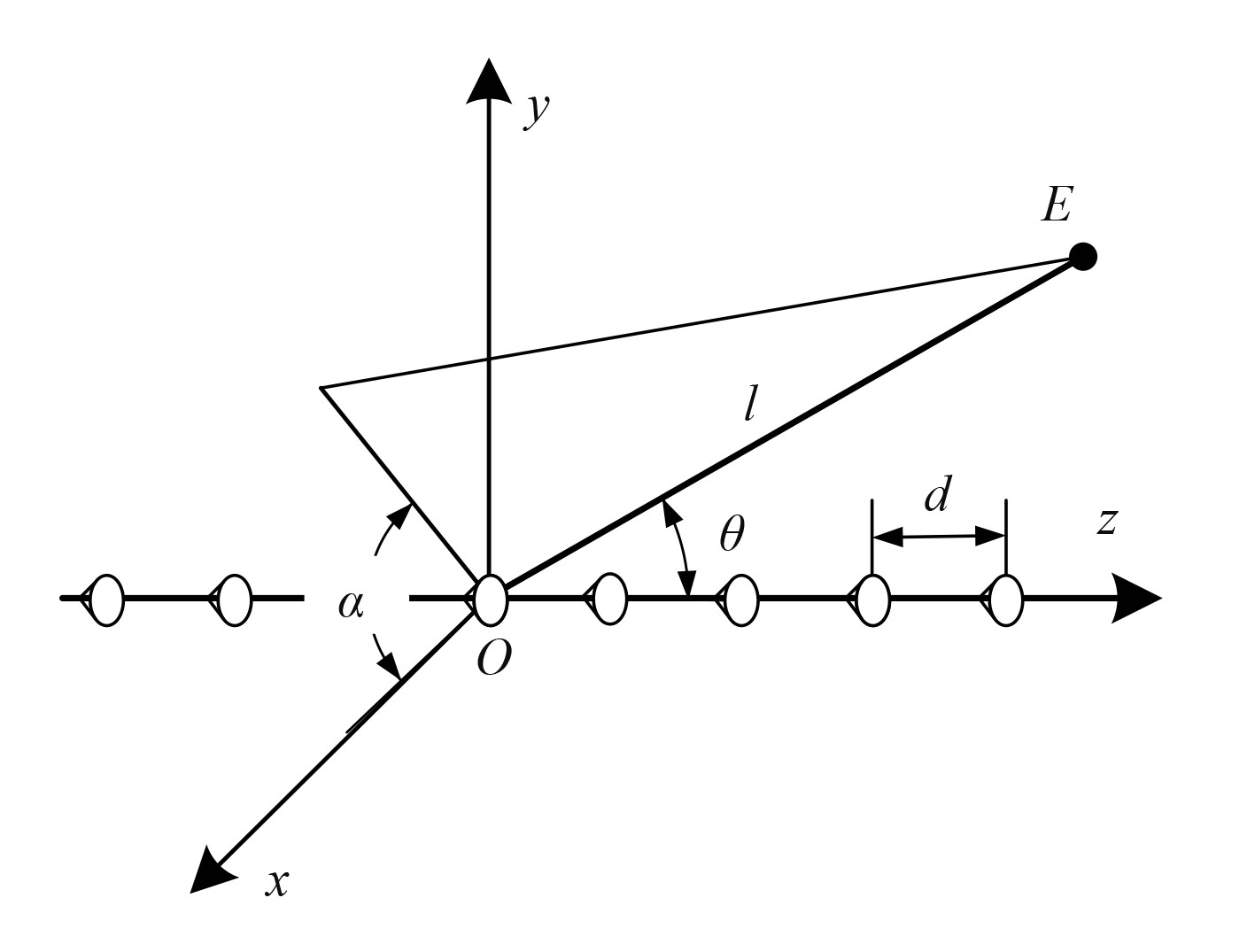图 1 线阵列阵元排列示意图 Fig. 1 Schematic diagram of line array arrangement
 ${{D}}{(\alpha ,\theta )_{{{piston}}}}{{ = }}\left| {\frac{{2{{{J}}_1}({{k}}a\cos \theta )}}{{ka\cos \theta }}} \right|,$ (1)

 ${{D}}{(\alpha ,\theta )_{{{linear}}}}{{ = }}\frac{{\sin (N\displaystyle\frac{{kd}}{2}\sin \alpha \cos \theta )}}{{N\sin (\displaystyle\frac{{kd}}{2}\sin \alpha \cos \theta )}},$ (2)

 \begin{aligned} {{D}}{(\alpha ,\theta )_{{N}}}={{D}}{(\alpha ,\theta )_{{{piston}}}} \times {{D}}{(\alpha ,\theta )_{{{linear}}}}& {{ = }}\\ \left| {\frac{{2{{{J}}_1}({{k}}a\cos \theta )}}{{ka\cos \theta }}} \right| \cdot \frac{{\sin (N\frac{{kd}}{2}\sin \alpha \cos \theta )}}{{N\sin (\frac{{kd}}{2}\sin \alpha \cos \theta )}} \end{aligned}。\text{。} (3)

1.2 移相电路设计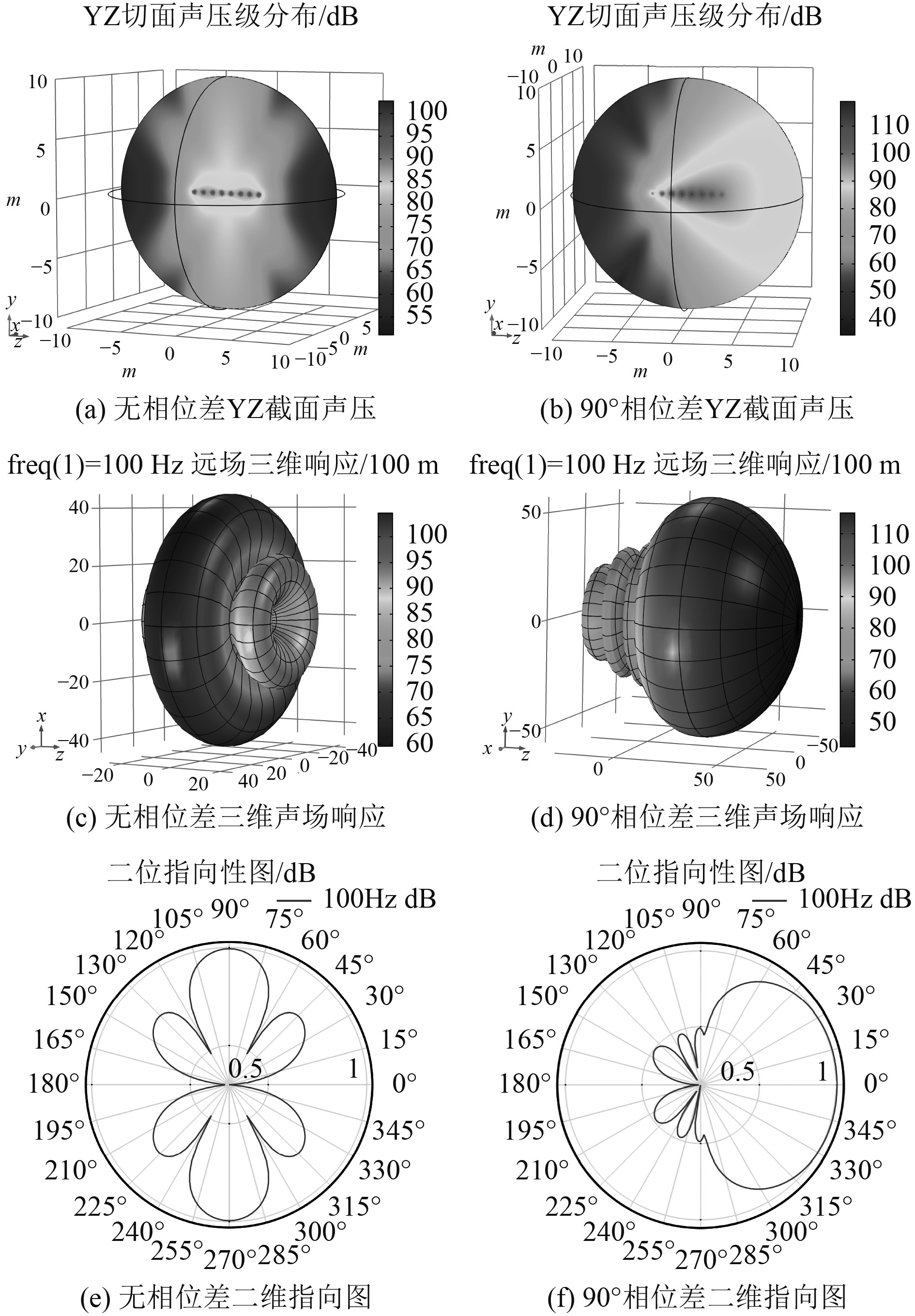图 2 8元线阵列设置相位差前后声场对比 Fig. 2 Comparison of sound field of 8-element line array under the condition of adjusting phase difference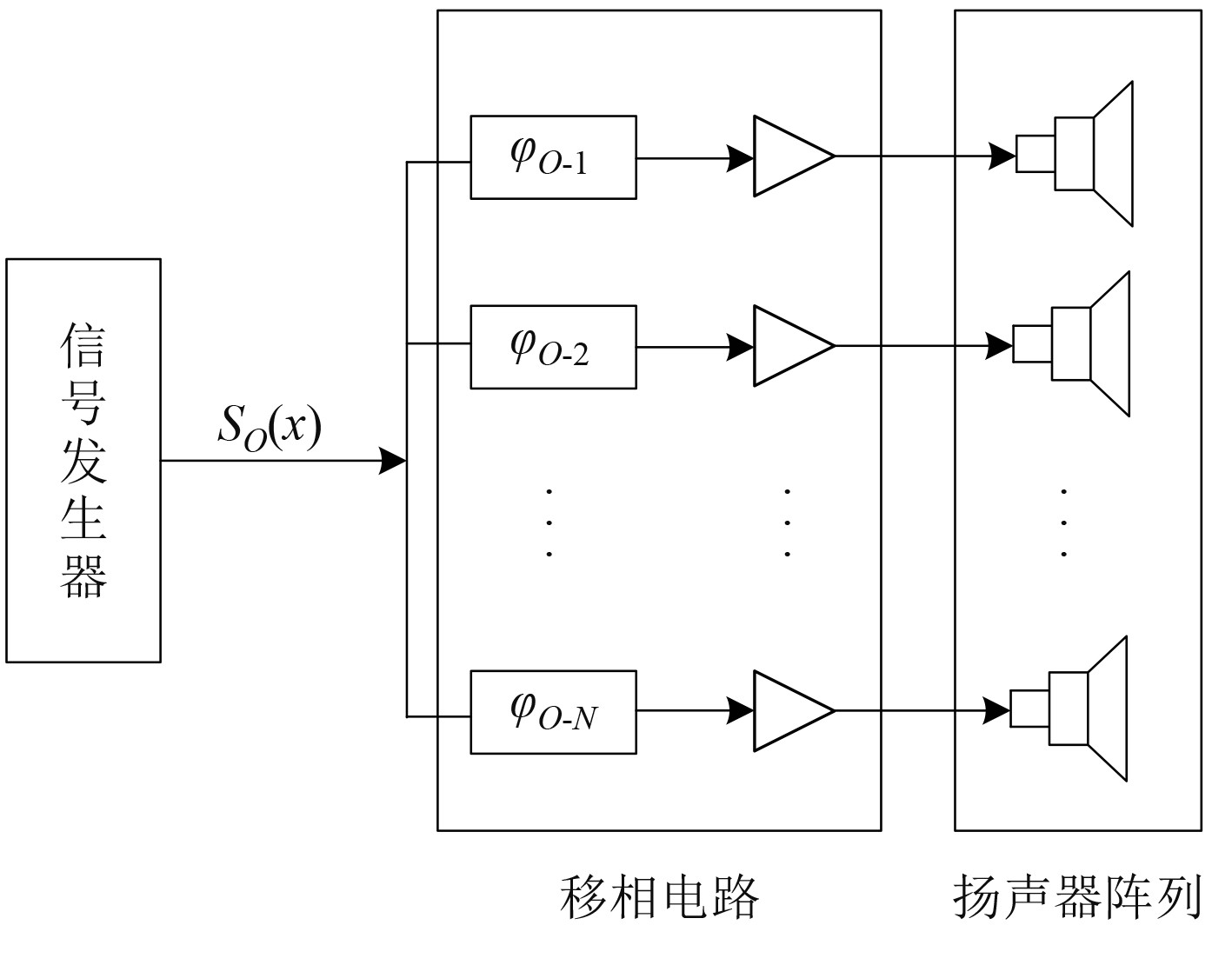图 3 移相电路工作示意图 Fig. 3 Schematic diagram of the phase shift circuit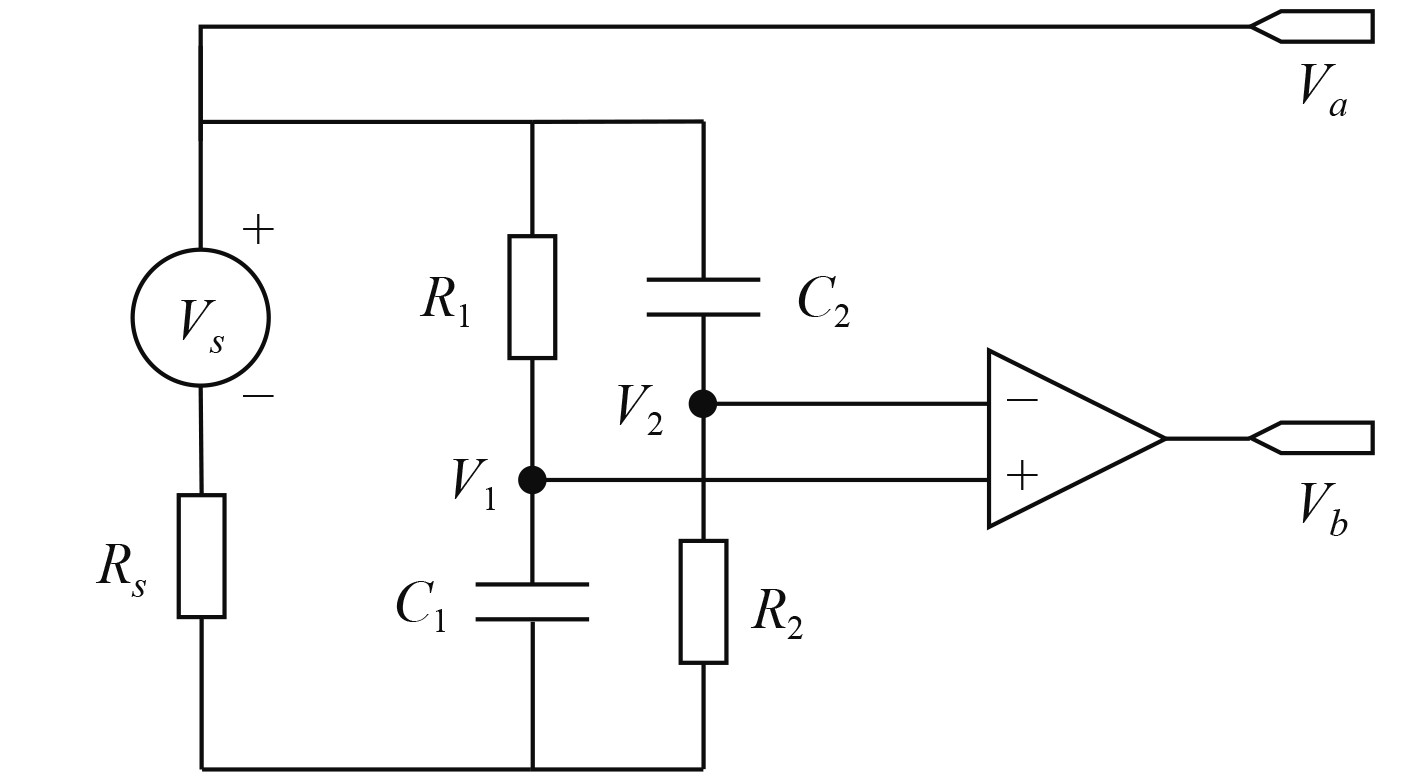图 4 全通桥式电路图 Fig. 4 All-pass bridge circuit

${C_{{1}}}{R_{{1}}} = {C_{{2}}}{R_{{2}}}$ ，分析得到 ${{{V}}_a},{V_{{b}}}$ 输出电压的关系为:

 $\begin{array}{l} {V_{\rm{b}}} = {V_{\rm{1}}} - {V_{\rm{2}}} = {V_{\rm{a}}}(\displaystyle\frac{1}{{1 + {{j}}\omega {C_{\rm{1}}}{R_{\rm{1}}}}} - \displaystyle\frac{{{{j}}\omega {C_{\rm{2}}}{R_{\rm{2}}}}}{{1 + {{j}}\omega {C_{\rm{2}}}{R_{\rm{2}}}}})= \\ \begin{array}{*{20}{c}} {}&{ {V_{\rm{a}}}\angle 2\arctan (\omega {C_{\rm{1}}}{R_{\rm{1}}})}\text{。} \end{array} \end{array}$ (4)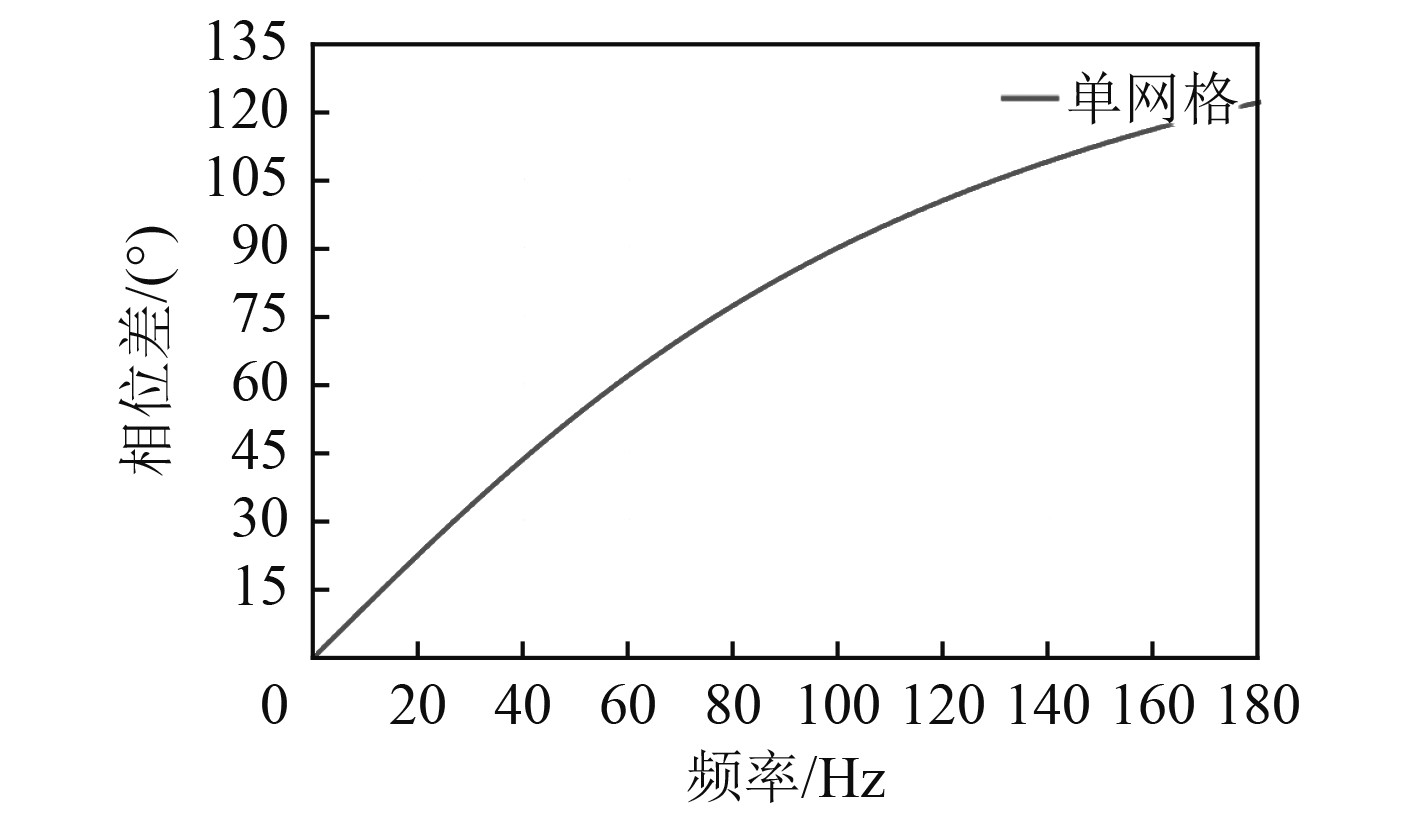图 5 单网络相位差随频率变化 Fig. 5 The variation of single network phase difference with frequency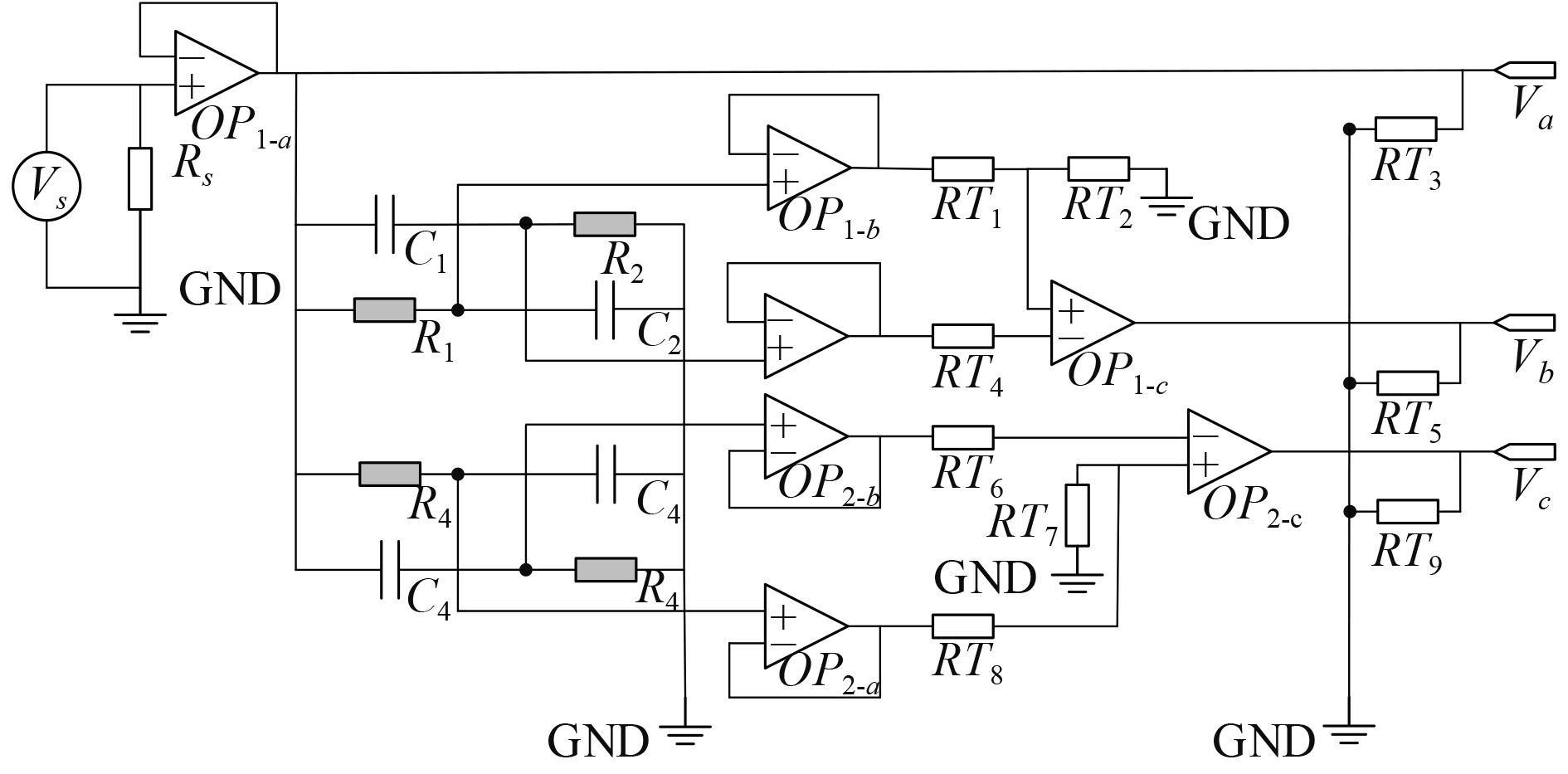图 6 双网络相位控制电路 Fig. 6 Dual network phase control circuit

${V_{\rm{b}}}$ ${V_{\rm{a}}}$ ${V_{\rm{c}}}$ ${V_{\rm{a}}}$ 的相位差分别为：

 \begin{aligned} & {\phi _{{{ba}}}} = 2\arctan (\omega {C_{{1}}}{R_{{1}}})\text{，} \\ & {\phi _{{{ca}}}} = 2\arctan (\omega {C_{{3}}}{R_{{3}}})\text{。} \\ \end{aligned} (5)

${C_{\rm{1}}} = {C_{\rm{2}}}{\rm{ = }}{C_{\rm{3}}} = {C_{\rm{4}}} = 1{\rm{\mu F}}$ ${R_{\rm{1}}} = {R_{\rm{2}}} = 659 \; \Omega$ ${R_3} = {R_4} = 3 \; 842 \; \Omega$ 。如图7所示，双移相网络对100 Hz附近频率， ${V_{\rm{b}}}$ ${V_{\rm{c}}}$ 的相位差 ${\phi _{{{cb}}}} = {\phi _{ca}} - {\phi _{ba}}$ 基本不变。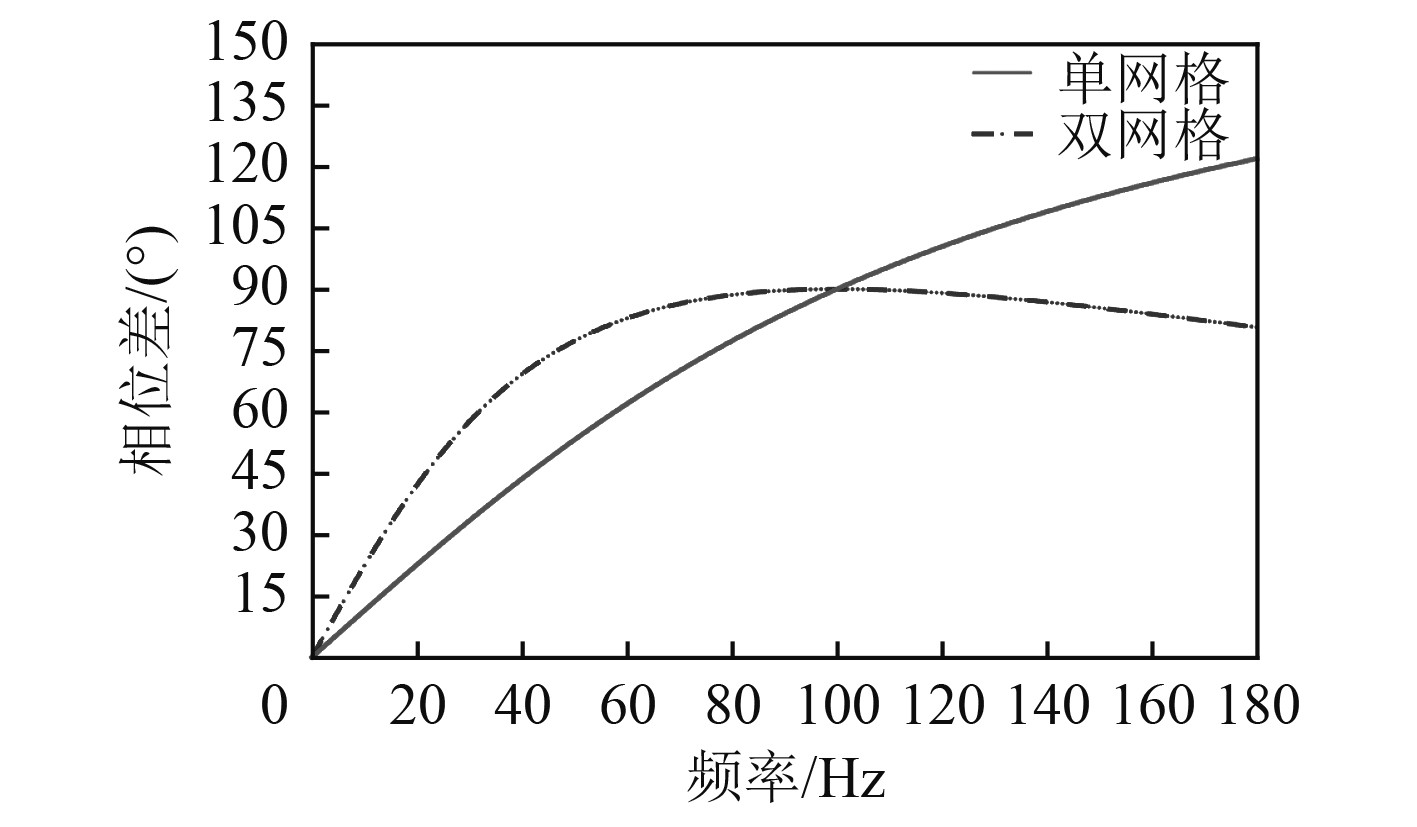图 7 单网络与双网络相位变化对比 Fig. 7 Comparison of phase change between single network and dual network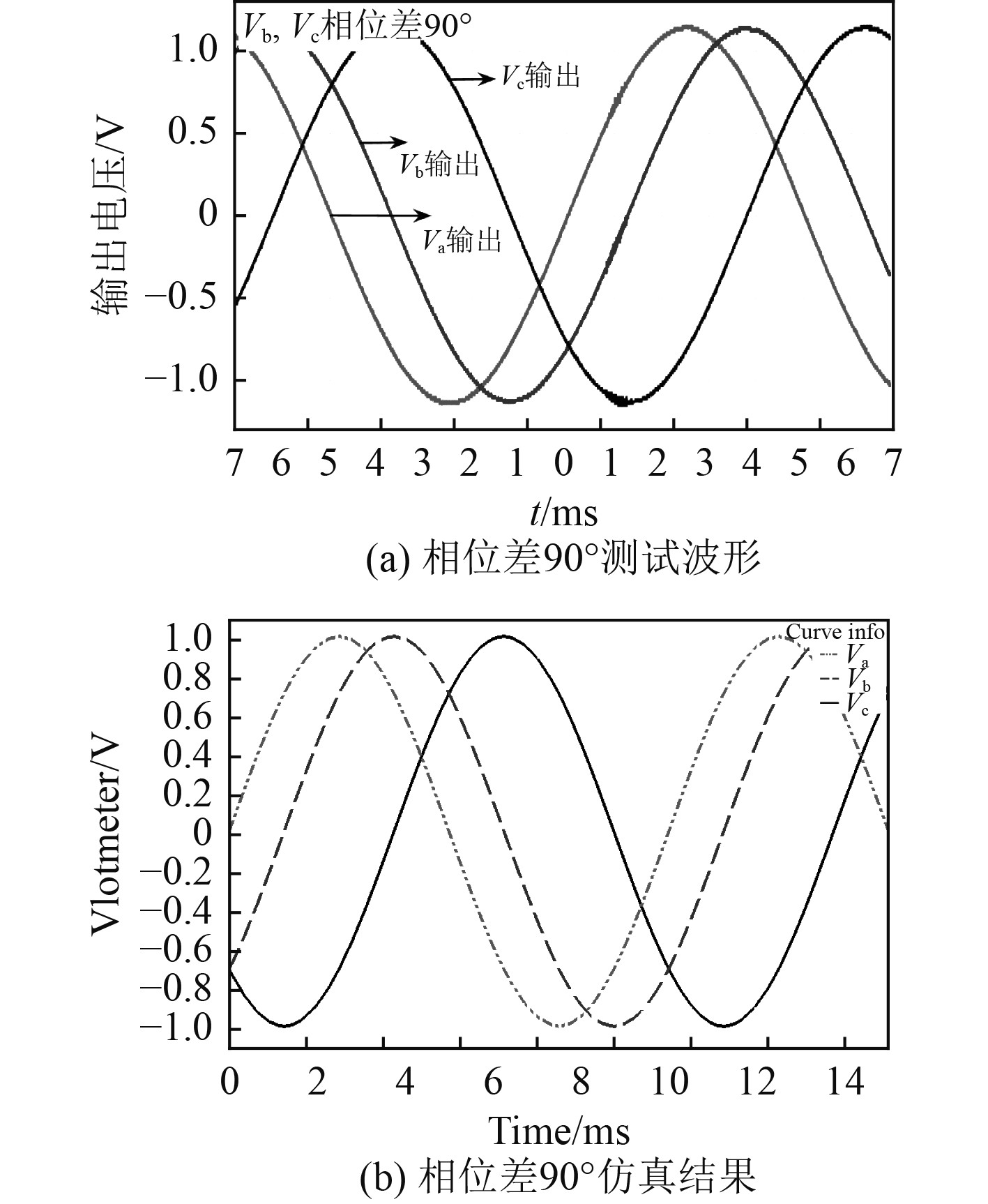图 8 移相电路测试与仿真结果 Fig. 8 Phase shift circuit test and simulation results
2 实　验表 1 扬声器产品参数 Tab.1 Speaker product parameters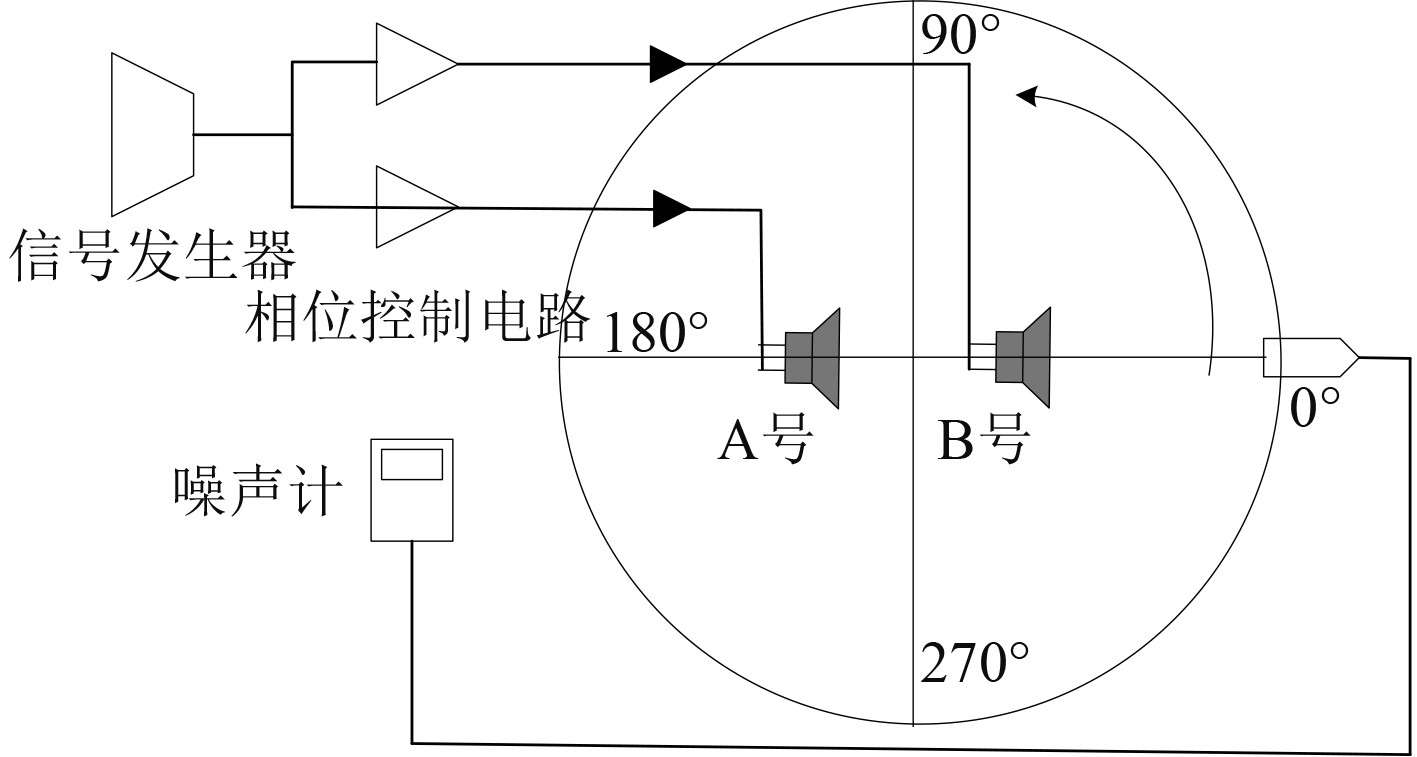图 9 双元扬声器指向性测试布置图 Fig. 9 Arrangement diagram of dual element loudspeaker directivity test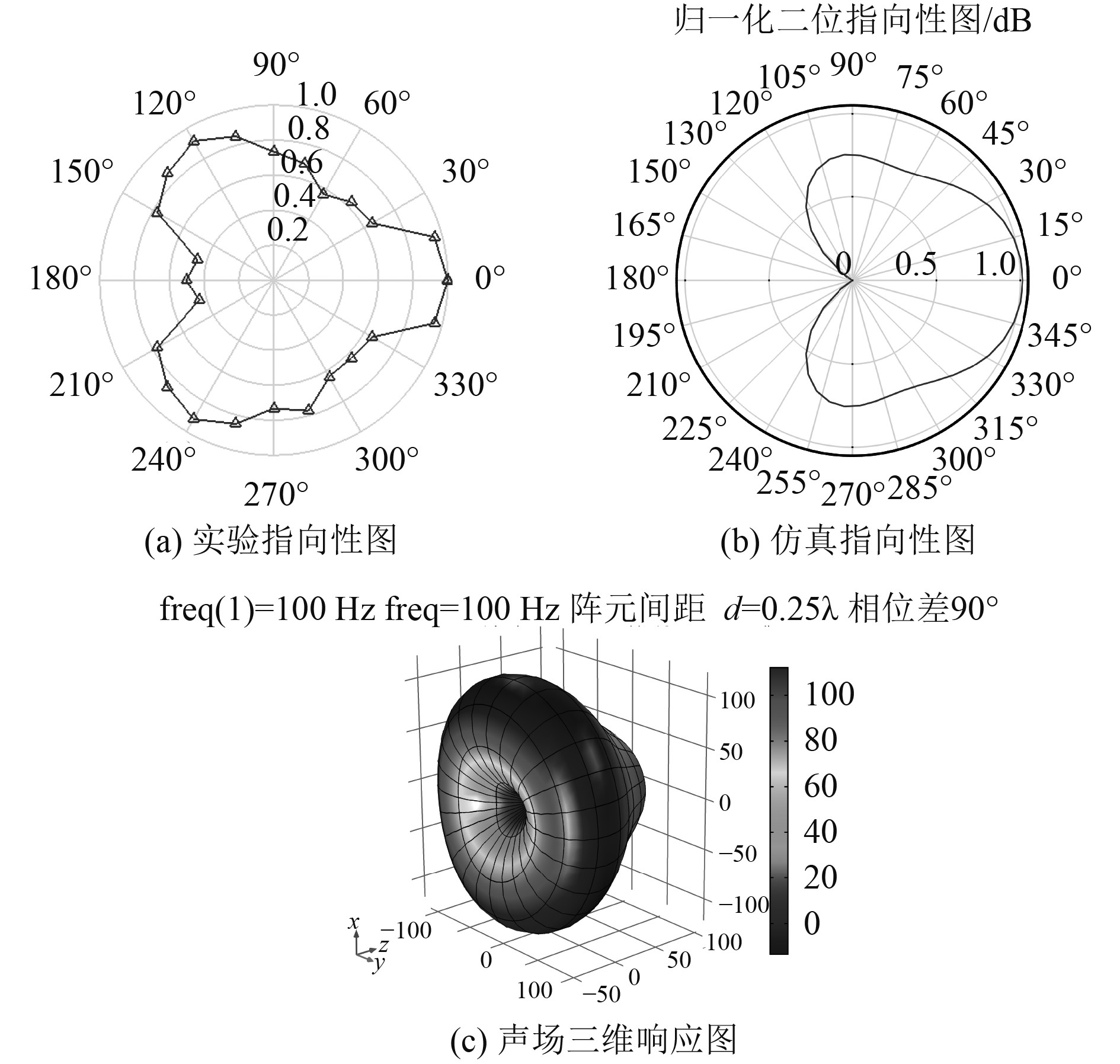图 10 双元扬声器合成声场分布情况 Fig. 10 The distribution of the sound field synthesized by the dual element speaker

3 结　语

  肖峰. 现场可控非致命的次声武器研究[J]. 兵工学报, 2002(03): 426-429. XIAO Feng. Research on on-site controllable non-fatal infrasound weapon[J]. Acta Armamentarii, 2002(03): 426-429. DOI:10.3321/j.issn:1000-1093.2002.3.037  张博, 梁延峰. 非火力打击武器发展研究[J]. 中国电子科学研究院学报, 2015, 10(1): 6-16. ZHANG Bo, LIANG Yan-feng. Research on the development of non-firepower combat weapons[J]. Journal of China Academy of Electronics and Information Technology, 2015, 10(1): 6-16. DOI:10.3969/j.issn.1673-5692.2015.01.002  蒋贤沛, 郭三学. 非致命声波武器综合效能评估研究[J]. 应用声学, 2016(2): 122-127. JIANG Xianpei, GUO Sanxue. Research on comprehensive effectiveness evaluation of non-fatal sonic weapons[J]. Applied Acoustics, 2016(2): 122-127.  张富东, 徐利梅, 陈敏. 相控参量阵波束合成设计[J]. 压电与声光, 2013, 35(2): 282-286. ZHANG Fudong, XU Limei, CHEN Min. Design of phase-controlled parametric array beam synthesis[J]. Piezoelectrics & Acoustooptics, 2013, 35(2): 282-286. DOI:10.3969/j.issn.1004-2474.2013.02.035  陈敏, 徐利梅, 黄大贵, 等. 基于MATLAB的换能器阵列指向性分析方法研究[J]. 电声技术, 2006(5): 25-28. CHEN Min, XU Limei, HUANG Dagui, et al. Research on directional analysis method of transducer array based on MATLAB[J]. Electroacoustic Technology, 2006(5): 25-28. DOI:10.3969/j.issn.1002-8684.2006.05.006  张亮永, 程章. 空气中相控阵声聚焦仿真研究[J]. 声学技术, 2017, 36(6): 79-82. ZHANG Liangyong, CHENG Zhang. Simulation of phased array sound focusing in Air[J]. Acoustic Technique, 2017, 36(6): 79-82.  杜功焕, 宋哲民. 声学基础[M]. 第三版. 南京: 南京大学出版社, 2012: 223-226.  周福洪. 水声换能器与基阵[M]. 北京: 国防工业出版社, 1984: 235-240.